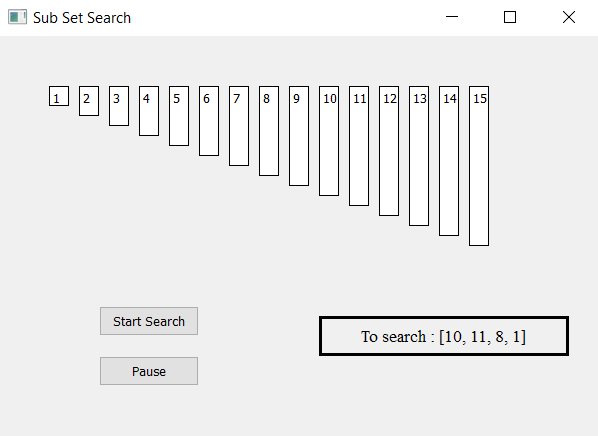Skip to content
Related Articles
Sub Set Search Visualizer using PyQt5
• Difficulty Level : Medium
• Last Updated : 06 May, 2020

In this article we will see how we can make a PyQt5 application which will visualize the subset search algorithm.

Sub Set Search : Sometimes we encounter the problem of checking if one list is just an extension of the list i.e just a superset of one list. This kind of problems are quite popular in competitive programming.

Examples –

Input: list = [1, 2, 3, 4, 5, 6, 7], sub-list = [2, 4, 7]

Output : List Found

Input: list = [1, 2, 3, 4, 5, 6, 7], sub-list = [5, 1, 7]
Output : List FoundGUI implementation steps :

1. Create a list of label according to the given list of numbers
2. Set their text, border, color and geometry with respective gap from each other
3. Each label height should be proportional to the value of each number
4. Create a start and pause push button to start the searching and pause the searching
5. Create a result label to show the searching status

Back end implementation steps :
1. Create label list corresponding to the given numbers
2. Create variable for the list index and sub-list index and flag for searching
3. Add action to the push button their action should change the flag status i.e start action should make flag true and pause action should make flag false and a counter to count number of matches
4. Create timer object which calls a method after every specific time
5. Inside the timer method check for the flag is flag is true begin the perfect sub-list search algorithm
6. Check for the first element of sub-list with first element of the list if found increment sub-list index, increment counter and reset the index of list else increment list index
7. If the index becomes equal to the list length stop the search and show result as not found.
8. If counter becomes equal to the length of the sub-list show result as found.

Below is the implementation

 `# importing libraries``from` `PyQt5.QtWidgets ``import` `*``from` `PyQt5 ``import` `QtCore, QtGui``from` `PyQt5.QtGui ``import` `*``from` `PyQt5.QtCore ``import` `*`` ` `import` `sys`` ` ` ` `class` `Window(QMainWindow):``    ``# list of numbers``    ``number ``=` `[``1``, ``2``, ``3``, ``4``, ``5``, ``6``, ``7``, ``8``, ``9``, ``                 ``10``, ``11``, ``12``, ``13``, ``14``, ``15``]`` ` `    ``# desired list``    ``desired ``=` `[``10``, ``11``, ``8``, ``4``]`` ` `    ``def` `__init__(``self``):``        ``super``().__init__()`` ` `        ``# setting title``        ``self``.setWindowTitle(``"Sub Set Search"``)`` ` `        ``# setting geometry``        ``self``.setGeometry(``100``, ``100``, ``600``, ``400``)`` ` `        ``# calling method``        ``self``.UiComponents()`` ` `        ``# showing all the widgets``        ``self``.show()`` ` `    ``# method for widgets``    ``def` `UiComponents(``self``):`` ` `        ``# start flag``        ``self``.start ``=` `False`` ` `        ``# list to hold labels``        ``self``.label_list ``=` `[]`` ` `        ``# match sub list``        ``self``.match ``=` `[]`` ` `        ``# index of both list``        ``self``.list_index ``=` `0``        ``self``.sub_index ``=` `0`` ` `        ``# local counter``        ``c ``=` `0`` ` `        ``# iterating list of numbers``        ``for` `i ``in` `self``.number:``            ``# creating label for each number``            ``label ``=` `QLabel(``str``(i), ``self``)`` ` `            ``# adding background color and border``            ``label.setStyleSheet("border : ``1px` `solid black;``                                 ``background : white;")`` ` `            ``# aligning the text``            ``label.setAlignment(Qt.AlignTop)`` ` `            ``# setting geometry using local counter``            ``# first parameter is distance from left ``            ``# and second is distance from top``            ``# third is width and forth is height``            ``label.setGeometry(``50` `+` `c ``*` `30``, ``50``, ``20``, i ``*` `10` `+` `10``)`` ` `            ``# adding label to the label list``            ``self``.label_list.append(label)`` ` `            ``# incrementing local counter``            ``c ``=` `c ``+` `1`` ` `        ``# creating push button to start the search``        ``self``.search_button ``=` `QPushButton(``"Start Search"``, ``self``)`` ` `        ``# setting geometry of the button``        ``self``.search_button.setGeometry(``100``, ``270``, ``100``, ``30``)`` ` `        ``# adding action to the search button``        ``self``.search_button.clicked.connect(``self``.search_action)`` ` `        ``# creating push button to pause the search``        ``pause_button ``=` `QPushButton(``"Pause"``, ``self``)`` ` `        ``# setting geometry of the button``        ``pause_button.setGeometry(``100``, ``320``, ``100``, ``30``)`` ` `        ``# adding action to the search button``        ``pause_button.clicked.connect(``self``.pause_action)`` ` `        ``# creating label to show the result``        ``self``.result ``=` `QLabel(``"To search : "` `+` `str``(``self``.desired), ``self``)`` ` `        ``# setting geometry``        ``self``.result.setGeometry(``320``, ``280``, ``250``, ``40``)`` ` `        ``# setting style sheet``        ``self``.result.setStyleSheet(``"border : 3px solid black;"``)`` ` `        ``# adding font``        ``self``.result.setFont(QFont(``'Times'``, ``10``))`` ` `        ``# setting alignment``        ``self``.result.setAlignment(Qt.AlignCenter)`` ` `        ``# creating a timer object``        ``timer ``=` `QTimer(``self``)`` ` `        ``# adding action to timer``        ``timer.timeout.connect(``self``.showTime)`` ` `        ``# update the timer every 300 millisecond``        ``timer.start(``300``)`` ` `    ``# method called by timer``    ``def` `showTime(``self``):`` ` `        ``# checking if flag is true``        ``if` `self``.start:`` ` `            ``# if index exceeds limit``            ``if` `self``.list_index >``=` `len``(``self``.number):`` ` `                ``# stop the search and show result not found``                ``self``.start ``=` `False``                ``self``.result.setText(``"Not Found"``)``                ``return`` ` `            ``# check for the element``            ``if` `self``.desired[``self``.sub_index] ``=``=` `self``.number[``self``.list_index]:`` ` `                ``# append it the match counter``                ``self``.match.append(``self``.list_index)`` ` `                ``# make label color yellow and rest of whitee color``                ``for` `i ``in` `range``(``len``(``self``.label_list)):``                    ``self``.label_list[i].setStyleSheet(``                          ``"border : 1px solid yellow;"``                          ``"background-color : white;"``                                                     ``)``                     ` `                    ``# dor matched label make them yellow color``                    ``if` `i ``in` `self``.match:``                        ``self``.label_list[i].setStyleSheet(``                                 ``"border : 2px solid yellow;"``                                 ``"background-color : yellow;"` `)`` ` `                ``# increment sub index``                ``self``.sub_index ``+``=` `1`` ` `                ``# reset the list index``                ``self``.list_index ``=` `0`` ` `            ``# if not found``            ``else``:`` ` `                ``# make checked index label grey``                ``self``.label_list[``self``.sub_index ``+` `self``.list_index].setStyleSheet(``                                     ``"border :"` `"1px solid black;"``                                     ``"background-color : grey;"` `)`` ` `                ``# increment list index``                ``self``.list_index ``+``=` `1`` ` `            ``# if matches become equal to desired list``            ``if` `len``(``self``.match) ``=``=` `len``(``self``.desired):``                ``# stop search``                ``self``.start ``=``False`` ` `                ``# show result``                ``self``.result.setText(``"Found at : "` `+` `str``(``self``.match))`` ` `                ``# make matched index green``                ``for` `i ``in` `self``.match:``                    ``self``.label_list[i].setStyleSheet(``                            ``"border : 2px solid green;"``                            ``"background-color : lightgreen;"``)`` ` ` ` ` ` `    ``# method called by search button``    ``def` `search_action(``self``):`` ` `        ``# making flag true``        ``self``.start ``=` `True`` ` `        ``# showing text in result label``        ``self``.result.setText(``"Started searching..."``)`` ` `    ``# method called by pause button``    ``def` `pause_action(``self``):`` ` `        ``# making flag false``        ``self``.start ``=` `False`` ` `        ``# showing text in result label``        ``self``.result.setText(``"Paused"``)`` ` ` ` `# create pyqt5 app``App ``=` `QApplication(sys.argv)`` ` `# create the instance of our Window``window ``=` `Window()`` ` `# start the app``sys.exit(App.``exec``())`

Output :

Attention geek! Strengthen your foundations with the Python Programming Foundation Course and learn the basics.

To begin with, your interview preparations Enhance your Data Structures concepts with the Python DS Course. And to begin with your Machine Learning Journey, join the Machine Learning – Basic Level Course

My Personal Notes arrow_drop_up